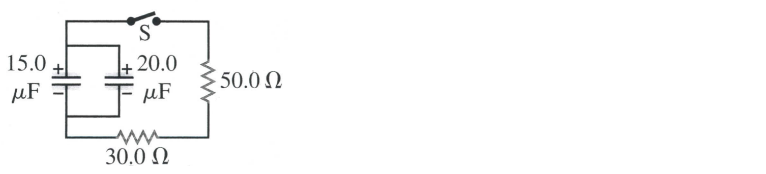# Problem: In the circuit shown below, both capacitors are initially charged to 45.0 V. How long after closing the switch S will the potential across each capacitors be reduced to 10.0 V? Determine the current through the resistors when the capacitors have a potential difference of 10.0 V.

###### FREE Expert Solution
90% (380 ratings)
###### Problem Details

In the circuit shown below, both capacitors are initially charged to 45.0 V. How long after closing the switch S will the potential across each capacitors be reduced to 10.0 V?

Determine the current through the resistors when the capacitors have a potential difference of 10.0 V.Frequently Asked Questions

What scientific concept do you need to know in order to solve this problem?

Our tutors have indicated that to solve this problem you will need to apply the !! Resistor-Capacitor Circuits concept. If you need more !! Resistor-Capacitor Circuits practice, you can also practice !! Resistor-Capacitor Circuits practice problems.

How long does this problem take to solve?

Our expert Physics tutor, Juan took 7 minutes and 29 seconds to solve this problem. You can follow their steps in the video explanation above.

What professor is this problem relevant for?

Based on our data, we think this problem is relevant for Professor Velissaris' class at UCF.